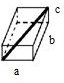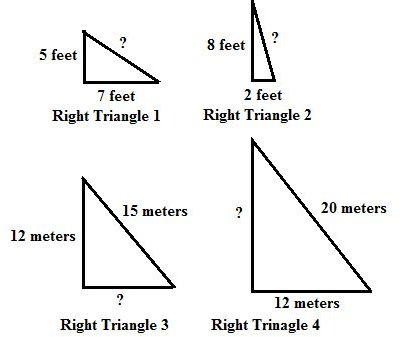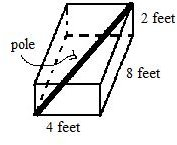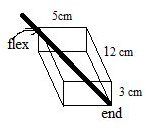# Practice Using the Pythagorean Theorem to Find Missing Sides of Right Triangles: Grade 8 Lesson Plan

Page content

Use the Pythagorean Theorem to solve each of the problems.

Learning Objective: The lesson is aligned to the Common Core State Standards for Mathematics - 8.G.7 Geometry - Apply the Pythagorean Theorem to determine unknown side lengths in right triangles in real-world and mathematical problems in two and three dimensions.

Materials Required: graph paper, calculator

## Lesson Procedure

**

Two-Dimensional Figures

1. The triangle shown here is a right triangle.
2. Side a equals 10 meters.
3. Side b equals 6 meters.
4. Use the Pythagorean Theorem a2 + b2 = c2, to find the length of side c of the right triangle. Calculate the length to the nearest tenth.

Three Dimensional Figures

1. ****The dimensions of a rectangular box are 4 cm x 5 cm x 3 cm. What is the length of the longest pole you could fit in the rectangular storage unit?

2. Let a = 4 cm, b = 5 cm, and c = 3 cm.

3. Use the formula = + + , to find the length of the longest pole (x). Calculate the length to the nearest tenth.

## Individual or Group Work:

Questions 1 - 4. Triangles drawn below are right triangles. Find the missing length for each right triangle. Calculate the length to the nearest tenth.5. The dimensions of a rectangular storage unit are 4ft x 8ft x 2ft. What is the length of the longest pole you could fit in the rectangular storage unit? Calculate the length to the nearest tenth.6. The dimensions of a rectangular juice box are 12 cm x 5 cm x 3 cm. What is the maximum length of a straw from the flex part of the straw to the end of the straw that can be used in the juice box? Calculate the length to the nearest tenth.Students should be able to apply the Pythagorean Theorem, a2 + b2 = c2 to determine unknown side lengths in right triangles in real-world problems with two-dimensional and three-dimensional figures.